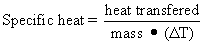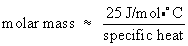Determining identity of metal by thermodynamic properties

Purpose: To determine the specific heat, and estimate the molecular weight of an unknown metal.

The specific heat of a substance is defined as the amount of heat energy required to change the temperature of one gram of a substance one degree Celsius. In the SI system, heat energy, as well as other forms of energy, is expressed in joules (J). Specific heat may be determined experimentally by measuring the heat required to change the temperature of a given mass from temperature T1 to T2 (ΔT):A convenient way to determine the specific heat of a solid sample is to heat it in boiling water, and then place the sample into a known amount of cool water. Heat energy flows from the sample to the water and its container (the calorimeter), causing the temperature of the water and container to rise and the sample's temperature to fall. In this experiment, assume that the heat lost by the sample is absorbed by both the water and the calorimeter. Expressed mathematically,

-qmetal = qwater + qcalorimeter

where q represents a quantity of heat. There is a - sign in from of the heat of the metal, because heat lost by the metal = the amount of heat gained by the water and calorimeter.  Efficient calorimeters are expensive. You will use an inexpensive thermos instead of a conventional calorimeter. The error introduced, however, will not be large. In the lab, we will determine the heat capacity of your calorimeter before testing the metal sample.

With the metal sample, you will heat it up to a high tempertature and place it in a container of cool water, which will heat up as the metal cools. Knowing the initial and final temperature of the water we can determine the energy given off by the metal sample. Knowing the mass, we can then determine the specific heat of the sample.

We will then use the specific heat to approximate the molar mass of your unknown metal. This can be done with the “law” of Dulong and Petit. This “law” was developed in 1819 by French scientists Pierre Dulong and Alexis Petit, who suggested that a metal's specific heat was proportional to its molar mass. This relationship is only approximate, but it has become a useful rule of thumb. The so-called “law” of Dulong and Petit can be written asYou will use this “law” and your measured specific heat to discover the identity of the unknown metal.

Lab report: Two computer generated data tables that include ALL your measured data as well as calculated values. Below each table is a sample (well labeled!) of each calculation you did. Appropriate averages and standard devations are needed as well. Finally, a standard Short Form Memo commentary section.

Prelab question: (to be completed on a separate piece of recycled paper, handed to the instructor as you enter lab)

1. A sample of unknown metal has a mass of 36.7 g. This metal was placed in boiling water at 96.0oC until the metal had reached the temperature of the water. The metal was then placed in a foam cup with 50.0 g of water in it at 20.0oC. The heat capacity of the cup is 42.0 J/oC, and the temperature of the water went up to 24.0oC. What is the specific heat of the metal? HINT: - energy lost by the metal = heat absorbed by calorimeter + heat absorbed by water in calorimeter. ANOTHER HINT: watch your units! The specific heat for liquid water is 4.184 J/goC.

~MEO  Feb 2006